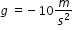# MA.912.C.3.8

Find average and instantaneous rates of change. Explain the instantaneous rate of change as the limit of the average rate of change. Interpret a derivative as a rate of change in applications, including velocity, speed and acceleration.

### Examples

Example: The vertical distance traveled by an object within the earth’s gravitational field, neglecting air resistance, is given by the equation x=0.5gt+ vot + xo, where g is the force on the object due to earth's gravity, vo is the initial velocity, xis the initial height above the ground, t is the time in seconds and down is the negative vertical direction. Determine the instantaneous speed and the average speed for an object, initially at rest, 3 seconds after it is dropped from a 100 m. tall cliff. Describe the object 5 seconds after it is dropped from the same height. Use.
General Information
Subject Area: Mathematics (B.E.S.T.)
Strand: Calculus
Status: State Board Approved

## Related Courses

This benchmark is part of these courses.
1202300: Calculus Honors (Specifically in versions: 2014 - 2015, 2015 - 2022, 2022 and beyond (current))

## Related Access Points

Alternate version of this benchmark for students with significant cognitive disabilities.

## Related Resources

Vetted resources educators can use to teach the concepts and skills in this benchmark.

## Perspectives Video: Expert

Velocity of the Aucilla River:

Harley Means discusses the mathematical methods hydrologists use to calculate the velocity of rivers.